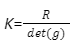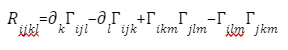# Exploring Intrinsic vs. Extrinsic Curvature in Differential Geometry

October 13, 2023Jackline Ross
United Kingdom
Differential Geometry
With a PhD in applied mathematics, Jackline Ross is one of the most adept assignment helpers. She has helped many students score good grades.

Differential geometry is a fascinating branch of mathematics that deals with the study of curves and surfaces in multidimensional spaces. One of the fundamental concepts in this field is the notion of curvature. Curvature allows us to understand how a surface curves and twists in space. In the realm of differential geometry, curvature is often categorized into two distinct types: intrinsic and extrinsic curvature. In this blog, we will delve deep into these two types of curvature and explore their significance in the mathematical world. Whether you are a university student looking to enhance your understanding of these concepts or seeking help to do your differential geometry assignment, this discussion will provide valuable insights.

## Intrinsic Curvature

Intrinsic curvature, also known as Gaussian curvature, is a property of a surface that is entirely dependent on the surface itself, without any reference to the ambient space in which it is embedded. In other words, intrinsic curvature is a geometric property that can be measured solely on the surface without considering the surrounding space.1. Gaussian Curvature
2. Gaussian curvature is a key aspect of intrinsic curvature. It measures how much a surface deviates from being flat at a given point. At any point on a surface, you can imagine a small circle centered at that point. Gaussian curvature tells you how the surface curves relative to this circle. There are three main possibilities for the sign of the Gaussian curvature:

• Positive Gaussian curvature: If the surface is locally shaped like a sphere, the Gaussian curvature is positive. Think of a sphere or a region of a saddle.
• Negative Gaussian curvature: If the surface is locally shaped like a hyperbolic surface, the Gaussian curvature is negative. Picture a saddle or a potato chip.
• Zero Gaussian curvature: If the surface is flat at a point, the Gaussian curvature is zero. This occurs when the surface is locally shaped like a plane.
3. Theorema Egregium
4. One of the most remarkable results in the theory of intrinsic curvature is the Theorema Egregium (Latin for "remarkable theorem"), established by Carl Friedrich Gauss. This theorem states that Gaussian curvature is an intrinsic invariant, meaning it remains constant even if you bend or stretch the surface without tearing or gluing it. In essence, you can determine the intrinsic geometry of a surface just by measuring its Gaussian curvature, regardless of how it is embedded in three-dimensional space.

## Extrinsic Curvature

Extrinsic curvature, in contrast to intrinsic curvature, depends on how the surface is situated within the surrounding space. It is a measure of how the surface curves within the ambient space in which it is embedded. Extrinsic curvature is essential when you want to understand how a surface interacts with its environment.

1. Normal Vector and Principal Curvatures
2. To study extrinsic curvature, we introduce the concept of a normal vector at each point on the surface. The normal vector points perpendicular to the surface and is a crucial element in understanding how the surface curves within space. Principal curvatures are the maximum and minimum curvatures of the surface along the directions given by the normal vector. These principal curvatures provide information about the rate of bending of the surface in different directions.

3. Mean Curvature and Shape Operators
4. Mean curvature is an extrinsic measure that quantifies the average curvature of the surface at a particular point. It is computed as the average of the principal curvatures and is a crucial parameter in various geometric applications. Additionally, the shape operator, a linear transformation, relates the normal vectors and principal curvatures, providing a comprehensive description of the extrinsic curvature.

## Intrinsic vs. Extrinsic Curvature: A Comparative Analysis

Intrinsic vs. extrinsic curvature is a fundamental concept in differential geometry, offering unique insights into the geometry of surfaces. Understanding their differences is essential for solving complex mathematical problems, making informed decisions in various fields, and unlocking the profound beauty of curvature in mathematics and the natural world.

• Independence: The distinction between intrinsic and extrinsic curvature lies in their dependence on the surrounding space. Intrinsic curvature, encapsulated by Gaussian curvature, is solely reliant on the surface itself, making it an intrinsic invariant. This independence from external factors enables mathematicians to explore the inherent geometric properties of surfaces, like the spherical geometry of a sphere or the hyperbolic geometry of a saddle. Extrinsic curvature, conversely, is intimately tied to the ambient space, highlighting how surfaces interact with their environment. This duality offers a rich perspective for mathematicians, physicists, and engineers alike, allowing them to tackle a myriad of real-world challenges from different angles.
• Measurement: Measurement is a pivotal aspect of distinguishing between intrinsic and extrinsic curvature in differential geometry. Intrinsic curvature, encapsulated by Gaussian curvature, is quantified purely within the surface itself, reflecting how it bends or stretches locally. This self-contained measurement allows mathematicians to analyze and characterize the geometry of surfaces independently of their embedding in a larger space. On the contrary, extrinsic curvature relies on measurements that encompass the surrounding space and involves factors like normal vectors and principal curvatures. Understanding this crucial distinction empowers students and researchers to apply the appropriate curvature concepts in various mathematical, scientific, and engineering contexts, enriching their problem-solving capabilities.
• Applications: Applications of intrinsic and extrinsic curvature extend into numerous domains, making them invaluable concepts in practical settings. In physics, intrinsic curvature aids in the study of gravity's effects on spacetime, while extrinsic curvature plays a role in modeling how surfaces bend under external forces. Engineers use curvature principles to design efficient structures and materials, and architects leverage them to create iconic buildings with curved surfaces. In the realm of computer graphics and animation, understanding curvature helps render lifelike 3D environments. Moreover, these concepts are crucial in fields like cartography for accurate map projections and in robotics for path planning on uneven terrains.
• Theorema Egregium: The Theorema Egregium, a Latin term for "remarkable theorem," is a cornerstone of differential geometry. It was formulated by Carl Friedrich Gauss and stands as a testament to the intrinsic elegance of mathematics. This theorem's brilliance lies in its revelation that Gaussian curvature, a key measure of intrinsic curvature, remains unchanged under bending or stretching of a surface, provided there is no tearing or gluing. In essence, it emphasizes that we can capture a surface's intrinsic geometry simply by examining its curvature properties, completely independent of how it may be embedded in a larger space. The Theorema Egregium continues to inspire mathematicians and students alike, highlighting the profound beauty and depth of mathematical concepts.

## Differential Geometry and the Study of Curvature

To delve deeper into the world of differential geometry and curvature, it's essential to understand the tools and concepts used in this field. One such tool is the notion of a metric, which defines how distances are measured on a surface. The metric tensor, denoted as g, plays a pivotal role in the study of curvature.

1. The Metric Tensor
2. The metric tensor, g, is a mathematical object that encodes information about the geometry of a surface. It defines the inner product between tangent vectors at each point on the surface, allowing us to measure angles and distances locally. In the context of differential geometry, the metric tensor is used to compute the lengths of curves on a surface and, importantly, to define the notion of angles and orthogonality.

For intrinsic curvature, the metric tensor is a crucial tool because it helps calculate the Gaussian curvature at each point. The Gaussian curvature, denoted as K, can be expressed in terms of the metric tensor components as follows:where R is the determinant of the Riemannian curvature tensor, a measure of the curvature of the space in which the surface is embedded. This formula highlights the connection between intrinsic curvature (Gaussian curvature) and the geometry of the surface itself (represented by the metric tensor).

3. Riemannian Curvature Tensor
4. The Riemannian curvature tensor, often denoted as R, captures the intrinsic curvature of a space. It describes how the metric tensor changes as one moves along different directions on the surface. In essence, it measures the deviation from Euclidean geometry.

The components of the Riemannian curvature tensor are given by:where ij kare the Christoffel symbols, which represent the connection between tangent vectors on the surface.

Understanding the Riemannian curvature tensor is crucial for students grappling with differential geometry assignments. It enables them to compute intrinsic curvature properties and make meaningful geometric interpretations of surfaces.

## Applications in Mathematics Assignments

Understanding intrinsic and extrinsic curvature is crucial for university students dealing with math assignments in various fields. Here are a few ways in which this knowledge can be applied to solve mathematical problems:

1. Differential Geometry Problems: When tasked with solving problems related to surfaces, knowing the distinction between intrinsic and extrinsic curvature can guide your approach. Gaussian curvature and principal curvatures often appear in these problems.
2. Topological Analysis: Intrinsic curvature plays a vital role in topological studies. Students can use it to determine whether two surfaces are topologically equivalent or to calculate the Euler characteristic of a surface.
3. Physics and Engineering: Extrinsic curvature is essential in applications related to physics, such as the study of gravitational forces on curved surfaces, and in engineering, where understanding the behavior of materials on curved surfaces is crucial.

## Real-World Applications

Differential geometry, with its intrinsic and extrinsic curvature concepts, finds applications beyond mathematics and into various scientific and engineering disciplines. Here are a few examples:

• General Relativity: Albert Einstein's theory of general relativity relies heavily on the principles of differential geometry. The curvature of spacetime, as described by the Einstein field equations, is a manifestation of intrinsic curvature. The presence of massive objects curves the spacetime around them, affecting the paths of particles and light.
• Cartography: In cartography, the science of mapmaking, understanding curvature is crucial. Cartographers use differential geometry to project the curved Earth's surface onto flat maps accurately, taking into account distortions caused by this projection.
• Robotics: Robot path planning often involves navigating curved surfaces. Knowledge of extrinsic curvature helps in designing robots that can move efficiently and safely on complex terrains.
• Computer Graphics: Computer graphics professionals use concepts of curvature to create realistic 3D models of surfaces. Rendering software relies on these principles to simulate the way light interacts with curved surfaces.
• Architecture: Architects use curvature concepts when designing buildings with curved surfaces. Differential geometry helps in achieving both aesthetically pleasing and structurally sound designs.

## Conclusion

Intrinsic and extrinsic curvature are fundamental concepts in differential geometry, each with its unique characteristics and applications. Intrinsic curvature, as exemplified by Gaussian curvature and the Theorema Egregium, provides a deep insight into the intrinsic properties of a surface. Extrinsic curvature, on the other hand, deals with how a surface interacts with its surrounding space, making it indispensable in various practical applications. Whether you are a student aiming to do your math assignment or simply curious about the intricate world of differential geometry, mastering these concepts will undoubtedly enhance your mathematical toolkit. So, as you embark on your journey through the fascinating realm of differential geometry, remember to keep the distinction between intrinsic and extrinsic curvature in mind, as it will serve as a valuable guide in solving math assignments and gaining a deeper appreciation for the mathematical beauty that lies within the curves and surfaces of our world.